# Fundamentals of Physics - high school - math problems

#### Number of problems found: 136

• Two forces 3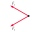Two forces of magnitude 8 Newtons and 15 Newtons respectively act at a point. If the resultant force is 17 Newtons, find the angle between the forces.
• At what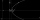At what speed did the motorist drive when he reacted by noticing the obstacle by 0.8 s; the magnitude of the opposite acceleration during braking was 6.5 m/s ^ 2 and the car ran to a stop track 35 m?
• Manganin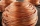How long must a manganin wire with a diameter of 0.8 mm have to have a value of 10Ω?
• The space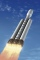The space rocket orbits the Earth at an altitude of 400 km above the Earth's surface. Determine the magnitude of the centrifugal acceleration of the rocket in its orbit.
• The satellite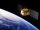The satellite orbiting the Earth at an altitude of 800 km has a speed of 7.46 km/s. For how long would it have to move from the start to the orbit to reach this speed if it evenly accelerated its motion in a straight line? What is the acceleration of sate
• Two waves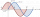Two waves are out of phase by 26°. If the period of the waves is 6 seconds, what is the time difference between the waves? Give your answer in seconds to 2 d. P.
• Angle ASB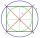On a circle with a radius of 10 cm and with a center S, the points A, B, C are given so that the central angle ASB is 60 degrees and the central angle ASC is 90 degrees. Find the length of the circle arc and the amount of AB and AC offsets.
• Ideal gas law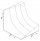Assuming compression is according to the law pV = constant. Calculate the initial volume of gas at a pressure of 2 bar which will occupy a volume of 6 cubic meters when it is compressed to a pressure of 42 bar?
• The projectile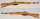The projectile leaves the 10 m long barrel at an instantaneous speed of 500 m/s. What is the acceleration of the movement of the projectile in the barrel and in what time will the projectile pass the barrel if, we assume that the movement was evenly accel
• Serial resistors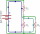In the circuit shown ( two resistiors R1 and R2 serial connected), the total resistance from A to B is 16 ohms. Find the resistance R2 and the current through the R1 = 12-Ohm resistor, if voltage between A and B is 10 V.
• Day trip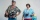A group of tourists went on a day trip. For two hours they walked at a speed of 6 km/h, for another hour they climbed a steep hill at a speed of 2 km/h, spent an hour and a half at lunch, then in an hour they inspected the castle garden at a free step at
• The oscillation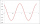The oscillation of a mechanical oscillator can be used to measure weight. A body of unknown weight is suspended on a spring with a stiffness of 20 N times m. The resulting oscillator oscillates with a frequency of 1.6 Hz. Find the weight of the body.
• Frequency of oscillation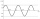A weight of 1 kg was suspended from the spring and the spring was extended by 1.5 cm. Determine the frequency of the natural oscillation of the resulting oscillator.
• Dynamometer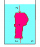What is the volume of a body that stretches a dynamometer in air, on which it is suspended by a force of 2.5 N, and if it is immersed in alcohol with a density of 800 kg/m ^ 3, does it tension the dynamometer with a force of 1.3 N?
• Above water surface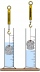If we remove the stone from the water, we apply a force of 120N. How much force will we have to exert if we move the stone above the water surface? The density of the stone is 5000 kg/m ^ 3.
• Two carsTwo cars (trucks and passenger) drove simultaneously from two places 50 km away. If they go against each other, they will meet in 30 minutes. If they follow each other in the same direction, the car will catch up with the truck in 2.5 hours. What is the s
• Two pendulums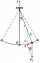In 24 s, the first pendulum performed 12 oscillations and the second 16 oscillations. Determine the periods and frequencies of both pendulums.
• Thermometer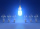Immerse a thermometer with a heat capacity of 2.0 J. K-1 in water weighing 67.0 grams. Before immersion in water, the thermometer showed a temperature of 17.8 degrees Celsius. After reaching equilibrium, the temperature is 32.4 degrees. What was the water
• Latent heat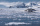How much heat is needed to take from 100 g of water at 20°C to cool to ice at -18°C? Mass heat capacity c (ice) = 21kJ/kg / °C; c (water) = 4.19 KJ/kg / °C and the mass group heat of solidification of water is l = 334kJ/kg
• The output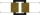The output voltage of the transformer is 880 V. The secondary coil has 1200 turns. Find the voltage to which the primary coil is connected and how many turns it has if a current of 1 A flows through it. Transformation ratio k = 4. What current flows throu

Do you have an exciting math question or word problem that you can't solve? Ask a question or post a math problem, and we can try to solve it.

We will send a solution to your e-mail address. Solved examples are also published here. Please enter the e-mail correctly and check whether you don't have a full mailbox.

Fundamentals of Physics - math problems. Examples for secondary school students.